https://doi.org/10.5194/acp-23-3195-2023
https://doi.org/10.5194/acp-23-3195-2023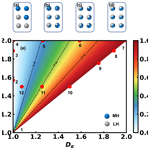# Quantifying particle-to-particle heterogeneity in aerosol hygroscopicity

Liang Yuan and Chunsheng Zhao
Abstract

The particle-to-particle heterogeneity in aerosol hygroscopicity is crucial for understanding aerosol climatic and environmental effects. The hygroscopic parameter κ, widely applied to describe the hygroscopicity for aerosols both in models and observations, is a probability distribution highly related to aerosol heterogeneity due to the complex sources and aging processes. However, the heterogeneity in aerosol hygroscopicity is not adequately represented in observations and model simulations, leading to challenges in accurately estimating aerosol climatic and environmental effects. Here, we propose an algorithm for quantifying the particle-to-particle heterogeneity in aerosol hygroscopicity, based on information-theoretic entropy measures, by using the data that come only from the in situ measurement of the hygroscopicity tandem differential mobility analyzer (H-TDMA). Aerosols in this algorithm are assumed to be simple binary systems consisting of the less hygroscopic and more hygroscopic components, which are commonly used in H-TDMA measurement. Three indices, including the average per-particle species diversity Dα, the bulk population species diversity Dγ, and their affine ratio χ are calculated from the probability distribution of κ to describe aerosol heterogeneity. This algorithm can efficiently characterize the evolution of aerosol heterogeneity with time in the real atmosphere. Our results show that the heterogeneity varies much with aerosol particle size, and large discrepancies exist in the width and peak value of particle number size distribution (PNSD) with varied heterogeneity after hygroscopic growth, especially for conditions with high relative humidity. This reveals a vital role of the heterogeneity in ambient PNSD and significant uncertainties in calculating the climate-relevant properties if the population-averaged hygroscopicity is applied by neglecting its heterogeneity. This work points the way toward a better understanding of the role of hygroscopicity in evaluating aerosol climatic and environmental impacts.

Share
Dates
1 Introduction

Aerosol hygroscopicity describes the interaction of aerosol particles and water vapor and severely influences aerosol climatic and environmental effects . It is highly related to the particle-to-particle heterogeneity in aerosol hygroscopicity, which is crucial but not adequately considered in observations and models, posing a challenge in accurately estimating aerosol effects on climate and environment.

Köhler theory (Köhler1936) is the basis of the studies on aerosol hygroscopicity. Nowadays, the most widely used parameter to describe aerosol hygroscopicity in observations and models is the hygroscopicity parameter, κ, proposed in κ-Köhler theory . κ extends and facilitates the application of Köhler theory and can be expediently observed under both subsaturated and supersaturated conditions . It can also be derived from the observed chemical composition . Considering that ambient aerosol particles in an aerosol differ dramatically in chemical composition due to the complex sources and aging processes , in terms of aerosol hygroscopicity, the distribution of the nearly hydrophobic (NH), the less hygroscopic (LH), and the more hygroscopic (MH) components vary between and within particles; such particle-to-particle heterogeneity in aerosol hygroscopicity results in a probability distribution of κ (κ-PDF) for an aerosol . Among the three abovementioned observing techniques, although the hygroscopicity tandem differential mobility analyzer (H-TDMA) can directly achieve κ-PDF under subsaturated condition, which is essential to investigate the hygroscopicity and activation property of aerosols, most studies focus on the analysis and application of the population-averaged κ (κmean) by neglecting the heterogeneity , leading to uncertainties in the estimation of aerosol impacts on climate and the environment.

Many research fields face the problem of heterogeneity involving the diversity of variables or issues. The diversity was first quantified in by introducing the information-theoretic entropy in ecology and then in many other fields, including economics (Drucker2013), immunology , neuroscience , and genetics . applied it in atmospheric science for the research of aerosol mixing states. By referring to and , the impact of the mixing state of black-carbon-containing (BC-containing) particles on light absorption enhancement was investigated, which showed that absorption is strongly affected by the heterogeneity of BC-containing aerosol and explained that the discrepancy between simulated and observed absorption enhancement accounts for the particle-to-particle heterogeneity in composition . How crucial is the heterogeneity in aerosol hygroscopicity for the highly climate-relevant aerosol size distribution and optical and activation properties? However, to the best of the authors’ knowledge, most studies are not focused on quantitatively evaluating the heterogeneity concerning aerosol hygroscopicity for in situ measurements.

Given these considerations, we proposed an algorithm for quantifying the particle-to-particle heterogeneity in aerosol hygroscopicity in the real atmosphere. This algorithm is based on information-theoretic entropy measures, employing observed data from the in situ measurement of the H-TDMA. In the following, Sect. 2 describes the algorithm in detail, and the interpretation of the results from the algorithm is presented in Sect. 3. The importance of the heterogeneity and the application of the algorithm are discussed and also included in Sect. 3. The last section comes to the conclusions.

2 Data and methods

## 2.1 Measurement site

The campaign was implemented for two periods from 23 January to 25 February and 19 July to 8 September 2019, respectively, at the meteorological station (30.58 N, 103.98 E) inside the campus of Chengdu University of Information and Technology (CUIT) located in the district of Shuangliu, southwest of the main urban area of Chengdu, China. The elevation of the observation site is approximately 500 m. Figure S1 in the Supplement shows the map of the site. It is surrounded by residential neighborhoods with no nearby sources of significant industrial pollution. Aerosol particles here are representative of the urban environments. More details can be found in .

## 2.2 Data of H-TDMA measurement

A custom-built H-TDMA designed by was employed to obtain the hygroscopic properties for aerosol particles with dry diameters Dp(Dry) of 40, 80, 110, 150, and 200 nm. The schematic structure of the H-TDMA is shown in Fig. S2. This H-TDMA was placed in a temperature-controlled (25 C) container. The aerosol inlet equipped with a PM2.5 impactor was set on top of the container (approximately 3 m aboveground). It mainly consists of two differential mobility analyzers (DMA) (Model 3081A, TSI Inc., USA), a humidification system, and a condensation particle counter (CPC) (Model 3787, TSI Inc., USA). Aerosol samples with a flow of 0.6 L  min−1 are first introduced into a Nafion dryer (Model MD-700-24S-3, Perma Pure Inc., USA) by an external vacuum pump to maintain the relative humidity (RH) below 10 %. These aerosol particles flow through an advanced aerosol X-ray neutralizer (Model 3088, TSI Inc., USA) to be single-charged and are then screened by the first DMA (DMA-1) with set voltages. Subsequently, the selected quasi-monodisperse dry particles feed into a Nafion humidifier (Model PD-07018T-24MSS, Perma Pure Inc., USA) with a RH of 90 % (±0.3 %). Finally, the PNSDs of humidified particles out of the humidifier are measured by the second DMA (DMA-2) coupled with the CPC. The details of the H-TDMA refer to .

The growth factor g of the quasi-monodisperse aerosol particles g[Dp(Dry)] selected by DMA-1 equipped in the H-TDMA can be then calculated by

$\begin{array}{}\text{(1)}& g\left[{D}_{\text{p}}\left(\text{Dry}\right)\right]=\frac{{D}_{\text{p}}\left(\text{RH}\right)}{{D}_{\text{p}}\left(\text{Dry}\right)},\end{array}$

where Dp(RH) represents the particle diameter screened by DMA-2 at a specific relative humidity (RH), e.g., 90 % in this study. The measured distribution function of g (g-MDF) is calculated from aerosol particle number size distribution (PNSD) counted by the CPC installed downstream of DMA-2. The TDMAfit algorithm is employed to convert the g-MDF to the actual probability distribution function (g-PDF) in the campaign . For each measured dry diameter, the g-PDFs are thereafter normalized as

$\begin{array}{}\text{(2)}& \underset{\mathrm{1}}{\overset{\mathrm{\infty }}{\int }}c\left(g\right)\text{d}g=\mathrm{1},\end{array}$

where c(g) represents the normalized g-PDF. For each g, κ can be converted using κ-Köhler theory :

$\begin{array}{}\text{(3)}& \mathit{\kappa }=\left({g}^{\mathrm{3}}-\mathrm{1}\right)×\left[\frac{\mathrm{1}}{\text{RH}}×\mathrm{exp}\left(\frac{\mathrm{4}{\mathit{\sigma }}_{s/a}{M}_{\text{w}}}{RT{\mathit{\rho }}_{\text{w}}{D}_{\text{p}}\left(\text{Dry}\right)g}\right)-\mathrm{1}\right],\end{array}$

where σs/a of 0.072 J  m−2 is the surface tension of the solution–air interface, Mw of 18.015 g  mol−1 is the molecular weight of water, ρw of 1.0 g  cm−3 is the density of water, R of 8.315 J  K−1 mol−1 is the universal gas constant, and T of 298.15 K is the temperature in Kelvin. Then, the κ-PDF can be obtained and normalized as

$\begin{array}{}\text{(4)}& \underset{\mathrm{0}}{\overset{\mathrm{\infty }}{\int }}c\left(\mathit{\kappa }\right)\text{d}\mathit{\kappa }=\mathrm{1},\end{array}$

where c(κ) is the normalized κ-PDF as the examples shown in Fig. 1.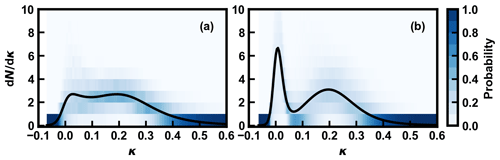Figure 1Typical κ-PDF for 100 nm aerosols in winter (a) and summer (b) measured by the H-TDMA over Chengdu. The shaded area is the frequency distribution of κ-PDF, and the solid lines show the campaign average of κ-PDF.

## 2.3 Calculation of the heterogeneity in aerosol hygroscopicity

The particle-to-particle heterogeneity in aerosol hygroscopicity is calculated by using the H-TDMA-measured κ-PDF (e.g., the examples in Fig. 1). The key assumption is that an aerosol containing N aerosol particles is a binary system consisting of the LH and MH components with respective κ of 0.01 (κLH) (typical for organics) and 0.6 (κMH) (typical for sulfate and nitrate). Each aerosol particle in the population contains one or two of the LH and MH components, and thus their κ varied between 0.01 and 0.6. κ-PDF of the aerosol can be considered the normalized aerosol number fractions varied with κ between 0.01 and 0.6 (Fig. 1). The volume fraction of these two components in each aerosol particle can be calculated based on κ according to the Zdanovskii–Stokes–Robinson (ZSR) mixing rule .

By referring to information-theoretic entropy measures , three indices, including the average per-particle species diversity Dα, the bulk population species diversity Dγ, and their affine ratio χ, are calculated to together describe the heterogeneity. The details are described as follows.

Firstly, for the aerosol with a given dry diameter and a known κ-PDF with X bins, the volume fraction of the LH and MH components at bin i ($i=\mathrm{1},\mathrm{2},\mathrm{3},\mathrm{\dots },X$), i.e., Pi,LH and Pi,MH, can be calculated by the combination of

$\begin{array}{}\text{(5)}& {P}_{i,\text{LH}}+{P}_{i,\text{MH}}=\mathrm{1}\end{array}$

and

$\begin{array}{}\text{(6)}& {P}_{i,\text{LH}}×{\mathit{\kappa }}_{\text{LH}}+{P}_{i,\text{MH}}×{\mathit{\kappa }}_{\text{MH}}={\mathit{\kappa }}_{i},\end{array}$

where κi is the κ at bin i.

Secondly, the mixing entropy for particles at bin i (Hi) can be calculated by

$\begin{array}{}\text{(7)}& {H}_{i}=-{P}_{i,\text{LH}}×\text{ln}{P}_{i,\text{LH}}-{P}_{i,\text{MH}}×\text{ln}{P}_{i,\text{MH}}.\end{array}$

Then the average particle mixing entropy for the aerosol (Hα) is calculated by

$\begin{array}{}\text{(8)}& {H}_{\mathit{\alpha }}=\sum _{j=\mathrm{1}}^{N}{P}_{j}×{H}_{j},\end{array}$

where Hj is the mixing entropy for particle j ($j=\mathrm{1},\mathrm{2},\mathrm{3},\mathrm{\dots },N$) in the aerosol. Pj is the volume proportion of particle j to the total volume of the aerosol, which is calculated by

$\begin{array}{}\text{(9)}& {P}_{j}=\frac{{V}_{j}}{{V}_{\text{total}}}=\frac{\mathrm{1}}{N},\end{array}$

because all particles have the same diameters. Considering that particles in the same κ bin have the same physical and chemical properties, they have the same mixing entropy. Equations (8) and (9) can be simplified as

$\begin{array}{}\text{(10)}& {H}_{\mathit{\alpha }}=\sum _{i=\mathrm{1}}^{X}{H}_{i}×c\left(\mathit{\kappa }{\right)}_{i}×\mathrm{\Delta }\mathit{\kappa },\end{array}$

where, c(κ)i is the probability density value of the normalized κ-PDF at bin i, and Δκ is the bin width.

Additionally, the population-level mixing entropy (Hγ) is calculated by

$\begin{array}{}\text{(11)}& {H}_{\mathit{\gamma }}=-{P}_{\text{LH}}×\text{ln}{P}_{\text{LH}}-{P}_{\text{MH}}×\text{ln}{P}_{\text{MH}},\end{array}$

where PLH and PMH are the respective volume fraction of the LH and MH components in the population, and they can be calculated by

$\begin{array}{}\text{(12)}& {P}_{\text{LH}}=\sum _{i=\mathrm{1}}^{X}{P}_{i,\text{LH}}×c\left(\mathit{\kappa }{\right)}_{i}×\mathrm{\Delta }\mathit{\kappa }\end{array}$

and

$\begin{array}{}\text{(13)}& {P}_{\text{MH}}=\sum _{i=\mathrm{1}}^{X}{P}_{i,\text{MH}}×c\left(\mathit{\kappa }{\right)}_{i}×\mathrm{\Delta }\mathit{\kappa }.\end{array}$

Thirdly, the per-particle species diversity (Di), the average per-particle species diversity (Dα), and the population species diversity (Dγ) can be calculated as

$\begin{array}{}\text{(14)}& {D}_{i}={e}^{{H}_{i}},\text{(15)}& {D}_{\mathit{\alpha }}={e}^{{H}_{\mathit{\alpha }}},\end{array}$

and

$\begin{array}{}\text{(16)}& {D}_{\mathit{\gamma }}={e}^{{H}_{\mathit{\gamma }}}.\end{array}$

Finally, a hygroscopic heterogeneity parameter χ can be calculated as

$\begin{array}{}\text{(17)}& \mathit{\chi }=\frac{{D}_{\mathit{\alpha }}-\mathrm{1}}{{D}_{\mathit{\gamma }}-\mathrm{1}},\end{array}$

which varied from 0 % that all particles in the population purely consist of the LH or MH component to 100 % that the LH and MH components are homogeneously distributed across all particles in the population with identical volume fractions.

## 2.4 Calculation of the size-resolved heterogeneity

A typical four-mode (i.e., a nucleation mode, an Aitken mode, an accumulation mode, and a coarse mode) PNSD with a wide size range of 3 nm–10 µm obtained by Ma (2013) is used for the calculation and presentation of the size-resolved heterogeneity. According to the assumption that aerosol particles in a specific mode have common sources or have experienced similar aging processes, the corresponding κmean and κ-PDF of one mode should be the same. The campaign average κmean and κ-PDF for particles with diameters of 40, 80, and 200 nm measured by the H-TDMA are used to deduce the respective κmean and κ-PDF for the nucleation mode, Aitken mode, and accumulation mode of the fitted PNSDs. Although the primary chemical compositions in the coarse-mode aerosol are nearly hydrophobic, some studies showed that this aerosol is slightly hygroscopic due to the coating in atmospheric aging processes . However, κ for the coarse-mode aerosol is hard to be observed by the H-TDMA technique so far, due to the technical bottleneck. Considering that this aerosol has limited influence on radiative forcing and cloud because of its short lifetime and low number concentration, κ for this aerosol is assumed to be 0 as set. According to the contribution of each mode to the κmean of specific particle size, the size-resolved κmean for aerosol particles ranging from 3 nm–10 µm can be estimated from the known κmean of each mode (κmean,i) as

$\begin{array}{}\text{(18)}& {\mathit{\kappa }}_{\text{mean}}\left({D}_{\text{p}}\right)=\frac{{\sum }_{i=\mathrm{1}}^{\mathrm{4}}{\mathit{\kappa }}_{\text{mean},i}×{N}_{i}\left({D}_{\text{p}}\right)}{{\sum }_{i=\mathrm{1}}^{\mathrm{4}}{N}_{i}\left({D}_{\text{p}}\right)}.\end{array}$

The size-resolved κ-PDF c(κ, Dp) can be calculated using the similar method with Eq. (19):

$\begin{array}{}\text{(19)}& c\left(\mathit{\kappa },{D}_{\text{p}}\right)=\frac{{\sum }_{i=\mathrm{1}}^{\mathrm{4}}{N}_{i}\left({D}_{\text{p}}\right)×c\left(\mathit{\kappa }{\right)}_{i}×\mathrm{\Delta }\mathit{\kappa }}{{\sum }_{i=\mathrm{1}}^{\mathrm{4}}{N}_{i}\left({D}_{\text{p}}\right)×\mathrm{\Delta }\mathit{\kappa }×\mathrm{\Delta }\text{log}{D}_{\text{p}}}.\end{array}$

Then, the size-resolved heterogeneity Dα(Dp), Dγ(Dp), and χ(Dp) for the wide range of 3 nm–10 µm can be obtained by using Eqs. (5)–(17).

3 Results

## 3.1 Diagram for particle-to-particle heterogeneity in aerosol hygroscopicity

From the calculation of Eqs. (15)–(17), Dα can represent the average of the effective number of species existing in each particle, and Dγ can represent the effective number of species in the population. Note that 1 $\le {D}_{\mathit{\alpha }}\le D\mathit{\gamma }$. Dα=1 when all particles are pure, while Dα=Dγ when all aerosol particles have identical components. The value of Dγ ranges from 1 to 2 according to the volume ratio of the LH and MH components in the population. Specifically, it is 1 when the population purely consists of the LH or MH component, while it is 2 when the population has the equivalent volume of the LH and MH components. A triangular region is thus presented in Fig. 2, in which χ is represented by contours.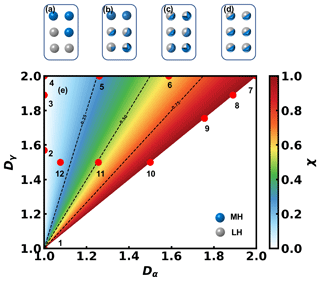Figure 2Example of the evolution of the heterogeneity in aerosol hygroscopicity (a–d) and diagram for the relationship among Dα, Dγ, and χ (e). Twelve different aerosols, each of which consists of six particles as shown in Table 1, are represented by the red points.

Table 1The sketch map of the components and the corresponding indices for the 12 different aerosols in Fig. 2. The LH and MH components are represented by the colors gray and blue, respectively; VLH and VMH represent their volumes.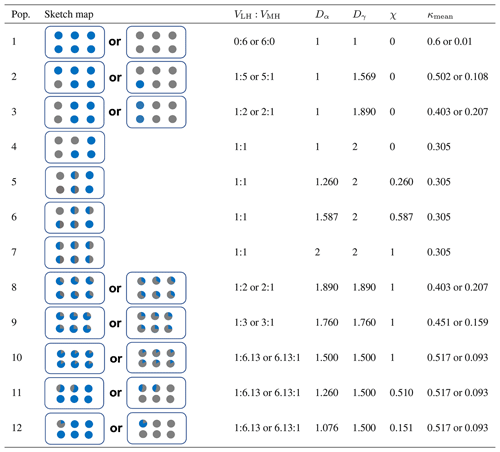Figure 2a–d show an example of the evolution of the heterogeneity in aerosol hygroscopicity. The distributions of the NH and MH components vary between and within populations, causing different heterogeneities for these aerosols. Twelve red dots in Fig. 2e represent aerosols, each consisting of six aerosol particles with varied fractions of the LH and MH components. Their respective sketch maps and heterogeneity indices are summarized in Table 1, and the corresponding κ-PDFs are listed in Fig. S3.

For aerosols with particles purely consisting of the LH component or MH component (e.g., populations 1–4 in Fig. 2e and Table 1), Dα equals 1 and χ equals 0, although Dγ varies from 1 to 2 due to the change of the bulk volume ratio of the LH and MH components. Their κ-PDFs show that κ distributes only at 0.01 for the LH component or 0.6 for the MH component due to the pure component in each particle.

For aerosols with equal bulk volume fractions of the LH and MH components (e.g., populations 4–7 and populations 10–12 in Fig. 2e and Table 1), Dγ have constant values of 2 and 1.5, respectively. However, the different distributions of the LH and MH components in each particle of an aerosol contribute to different Dα and χ. Specifically, the heterogeneous distribution (e.g., populations 4–6 and populations 11–12 in Fig. 2e and Table 1) leads to relatively small values of Dα and χ and the dispersed κ-PDF. On the contrary, the homogeneous distribution (e.g., populations 7 and 10 in Fig. 2e and Table 1) generates a narrow κ-PDF and thus relatively high Dα and χ.

For aerosols consisting of particles with identical volume fractions of the LH and MH components (e.g., populations 7–10 in Fig. 2e and Table 1), equal values of Dα and Dγ for each population result in χ of 1, although the bulk ratio of the LH to MH components changes between populations. Accordingly, their κ-PDFs are concentrated at a single value of κmean.

From the populations, it can be concluded that the particle-to-particle heterogeneity in hygroscopicity resulting from the distribution discrepancy of the LH and MH components within particles can be quantified according to the κ-PDF using the proposed algorithm of this work, which is of importance to the further analysis of the existing κ-PDF and can help the heterogeneity to be considered in the calculation of aerosol climate-relevant properties.

## 3.2 Evolution of the heterogeneity in the real atmosphere

In this section, we apply the proposed algorithm to the in situ measurement of the H-TDMA that was implemented for two periods in 2019, located in Chengdu, China. In the following, the expression of the κ-PDF is equivalent to the g-PDF due to their one-to-one corresponding relationship. In order to show the variation characteristics and the significance of Dα, Dγ, and χ in the real atmosphere, especially for the condition that the total hygroscopicity of aerosol remained constant, two episodes with a tiny change of κmean during the observation periods are presented. The 110 nm aerosol is chosen as an example to show the typical evolution processes for aerosol heterogeneity, because it has a large number concentration and lies in the transition zone between the diameters that show the processes of gas-to-particle (<100 nm) and aging (>100 nm) in the atmosphere. The variation of the heterogeneity in a 110 nm aerosol can better reflect the evolution of aerosol particles in the real atmosphere and may have a great impact on the direct radiative forcing. The first episode appeared from 22:00 (LST) 23 January 2019 to 08:00 (LST) 24 January 2019, and the other one occurred from 00:00 27 January 2019 to 12:00 27 January 2019. The variations of Dα, Dγ, χ, and κmean during these two episodes are shown in Fig. 3. The tiny change of κmean indicates the slight variation of the bulk fraction of the LH and MH components in the aerosol. The decreasing trends of Dα and χ reveal that the existing aged aerosols with uniformly distributed LH and MH components among particles at night, indicated by the unimodal distributions of κ-PDF and relatively high χ (0.831 for episode 1 at 22:00 and 0.832 for episode 2 at 00:00), were intruded upon by the fresh emissions during midnight as reflected by the bimodal and wider distribution of κ-PDF . This process led to component discrepancies among particles in populations and thus the decrease of Dα and χ during midnight.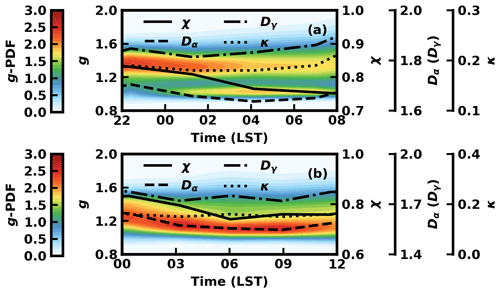Figure 3The variation of κ-PDF, Dα, Dγ, and χ during two typical evolution processes for 110 nm aerosols with tiny changes of κmean on 23 January 2019 (a) and 27 January 2019 (b).

The statistics of Dα, Dγ, and χ for aerosols with five measured diameters are shown in Fig. 4. The patterns of the distributions for Dα and Dγ move to larger values with increasing diameter, indicating that the effective number of species increases in aerosols with larger diameters due to the longer aging time in the atmosphere. This trend is more obvious in the summer campaign. Taking the aerosol of 110 nm for example, Dα varies between 1.386 and 1.850, and Dγ varies between 1.470 and 2.000 during the winter field measurements. The ranges of Dα and Dγ in summer are 1.280–1.928 and 1.371–2.000, respectively, contributing to a wider distribution of χ. This indicates that the fraction of the LH and MH components varied more pronouncedly due to the more complex aging processes in summer compared to that in winter. χ ranging from 0.6 to 0.9 reveals the large variation of heterogeneity in aerosol hygroscopicity in the real atmosphere.Figure 4The statistics of Dα, Dγ, and χ for aerosols of five measured diameters in winter (a)(b), (c) and summer (d)(e)(f). The shaded area is the frequency distribution of the indices. The three bars on the whisker for each diameter from the bottom to the top are the minimum, median, and maximum, and the circles represent the average of the indices.

The details in the variation characteristics of the heterogeneity and the difference among the aerosols of five measured diameters will be further discussed in our future studies. It can be concluded from the discussion above that aerosols with a tiny variation of κmean can have ever-changing heterogeneity in aerosol hygroscopicity in the atmosphere. The algorithm proposed in this work can efficiently characterize this heterogeneity with time and provide detailed information about the evolution of aerosols in the air. More importantly, this algorithm offers an unexplored understanding of the H-TDMA measurement.

## 3.3 Importance of the heterogeneity in aerosol hygroscopicity

The same bulk volume fraction of the LH and MH components indicates the same κmean for populations (e.g., populations 4–7 and populations 10–12). This reveals the identical diameter for all particles in each population after hygroscopic growth at high RH. Actually, due to the particle-to-particle heterogeneity in one population, different ratios of the LH and MH components in each particle result in different κ (e.g., particles in each of populations 4–7), which can lead to different ambient number size distributions, especially for the condition with high RH. Figure 5 depicts a sample of the hygroscopic size distribution, where RH is 90 %, for three aerosols with the same κmean of 0.305 but different χ of 0.653, 0.884, and 0.999, respectively. Each of these three populations contains 1000 aerosol particles with dry diameters of 100 nm. Significant discrepancies can be seen in the number size distribution that the width decreases, while the peak value increases with increasing χ after hygroscopic growth. Thus, the heterogeneity in aerosol hygroscopicity plays a significant role in the evolution of aerosol ambient number size distributions, which is a key factor for evaluating aerosol radiative forcing.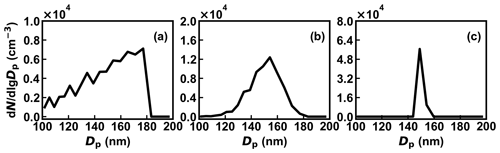Figure 5Aerosol particle size distributions after hygroscopic growth at a RH of 90 % for three aerosols with χ of 0.653 (a), 0.884 (b), and 1 (c). Each of these three populations has the same κmean of 0.305 and contains 1000 aerosol particles with dry diameters of 100 nm.

## 3.4 Size-resolved heterogeneity in aerosol hygroscopicity

The heterogeneity for only one size can contribute to dramatically different ambient size distributions, especially for the condition under high RH. Thus, it is urgently needed to figure out how the heterogeneity varies with aerosol size in the real atmosphere. This section discusses the characteristic of the size-resolved heterogeneity in aerosol hygroscopicity.

The PNSD within the range of 3 nm–10 µm is commonly observed by commercial instruments, including the scanning mobility particle sizer (SMPS) and the Wide-range Particle Spectrometer (WPS) around the world . However, the widely used H-TDMA technic can only observe the growth factor of aerosols with limited sizes (generally smaller than 350 nm in the dry condition), e.g., 40, 80, 110, 150, and 200 nm in this study. Recently, extended the H-TDMA measurement of aerosol hygroscopic properties to 600 nm in the urban environment. Although κ for larger particles (>1µm) can be derived from the size-resolved chemical composition , the size-resolved κ with high size and time resolution is scarce and is needed to be observed or reversed by more advanced technology and new algorithms. Fortunately, provided an approach to derive the size-resolved κ for aerosols within the range of 3 nm–10 µm based on the measured PNSDs and H-TDMA-determined κ. They used the κmean for each measured size by neglecting aerosol heterogeneity. Here, the heterogeneity in aerosol hygroscopicity is considered based on the method provided by .

The typical four-mode PNSD mentioned above is used and shown in Fig. 6a and d. The κmean and the corresponding κ-PDFs for the nucleation mode, Aitken mode, and accumulation mode are represented by those measured at sizes of 40, 80, and 200 nm, respectively, during the winter (Fig. 6b) and summer (Fig. 6e) campaigns. The calculated size-resolved κmean are shown in Fig. 6c (winter) and Fig. 6f (summer), in which κ-PDF for each size contributed by the heterogeneity in aerosol hygroscopicity is represented by the contour. The variation of both the size-resolved κmean and the corresponding κ-PDF is influenced by the contribution of each mode to the total number concentration. The corresponding χ for the size range of 3 nm–10 µm can therefore be calculated by using the framework proposed by this work.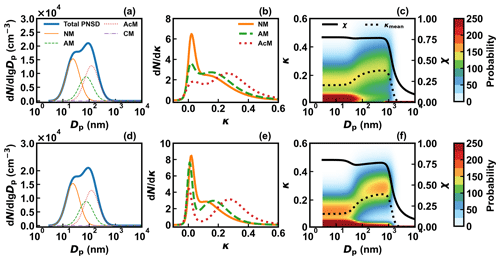Figure 6The typical PNSD (a), (d) and the κ-PDF during the winter (b) and summer campaigns (e) used for calculating the size-resolved κmean, the size-resolved κ-PDF, and the corresponding size-resolved χ (c), (f). The solid lines (blue) in (a) and (d) are the measured PNSD that can be fitted by a four-mode (a nucleation mode (NM), an Aitken mode (AM), an accumulation mode (AcM), and a coarse mode (CM)) lognormal distribution, which is represented by the thin solid line (orange), the dashed line (green), the dotted line (red), and the dash-dotted line (purple), respectively. The corresponding type and color lines represent the measured campaign average κ-PDF for each mode in (b) and (e). The dotted line in (c) and (f) is the calculated size-resolved κmean, and the shaded color is the corresponding size-resolved κ-PDF. The solid lines in (c) and (f) are the calculated size-resolved χ.

As shown in Fig. 6, the consideration of the heterogeneity provides the size-resolved κ-PDF, which can further show the region where κ is mostly distributed for aerosols of any size and how aerosol particles evolve with size in the population. For example, although the κmean for aerosols smaller than 40 nm is 0.131 during the winter campaign, the κ-PDF shows most κ locate at a narrow area lower than 0.05. The continuous evolution of the κ-PDF with sizes smaller than 1 µm indicates that on the one hand, a part of the aerosol particles in the population has increasing hygroscopicity during the aging process of growing up, which is the main reason for the increase of κmean, and on the other hand, NH-dominated particles exist across all sizes. This corresponds to the slight decreasing trend of χ accompanied by a wider area of κ ranging from 0.01 to larger than 0.4, which reflects the heterogeneous distribution of the LH and MH components between aerosol particles within a population during the aging progress.

Additionally, the phenomenon that a sharp decrease of χ close to 0.1 for aerosols larger than 1 µm and the κ-PDFs for this size range shows a very narrow band lying at κ of 0 responds to the assumption that the coarse-mode particle is mainly composed of the NH component.

Overall, this newly developed aerosol heterogeneity provides more insight into the evolution of aerosols during the aging processes. The hygroscopicity of an aerosol is complicated and diverse due to the particle-to-particle heterogeneity in aerosol hygroscopicity; if only the κmean is applied without considering aerosol heterogeneity, significant uncertainty will occur.

4 Conclusions

The particle-to-particle heterogeneity in aerosol hygroscopicity is of great importance for a better understanding of the impact of hygroscopicity on estimating aerosol climatic and environmental effects. Unfortunately, the heterogeneity has not been paid attention to in previous studies, of which only the population-averaged hygroscopicity parameter, κmean, is considered, mainly because the heterogeneity is difficult to be quantified in both observations and models.

In this work, we proposed an algorithm to quantify the particle-to-particle heterogeneity in aerosol hygroscopicity from the in situ measurement for the first time. This algorithm is an innovation on the basis of the mature theory in information-theoretic entropy and the widely used assumption that aerosols are binary systems consisting of the commonly defined LH and MH components from H-TDMA measurement.

Three indices, including the average per-particle species diversity Dα, the bulk population species diversity Dχ, and their affine ratio χ, are calculated from the probability distribution of κ to together describe aerosol heterogeneity. They can efficiently characterize and provide more insight into the evolution of aerosol heterogeneity with time in the real atmosphere during the aging processes.

The heterogeneity varies much with aerosol particle size, and large discrepancies can be seen in aerosol particle number size distribution (PNSD) that the width decreases, while the peak value of the PNSD increases with increasing χ after hygroscopic growth, especially for conditions with high relative humidity, indicating the vital role of the heterogeneity in the evolution of ambient PNSD.

Considering that PNSD is a key factor for the evaluation of aerosol impacts on radiative forcing, significant uncertainties will occur in calculating the climate-relevant properties if κmean is applied by neglecting its heterogeneity. Thus, the particle-to-particle heterogeneity in aerosol hygroscopicity is urgently needed to be represented in models. The algorithm proposed by this work, which has the intuitive and quantitative interpretation of aerosol heterogeneity, sheds light on the reanalysis of the existing H-TDMA datasets and could have a large impact on how we use and think about these datasets. It can also provide a bridge for using observations to constrain numerical models to deeply investigate how heterogeneity in aerosol hygroscopicity influences aerosol effects on climate and the environment.

Data availability

The data are available at https://doi.org/10.5281/zenodo.7320916 ().

Supplement

Author contributions

CZ designed the research project and reviewed the manuscript. LY interpreted all results and contributed to writing.

Competing interests

The contact author has declared that neither of the authors has any competing interests.

Disclaimer

Publisher’s note: Copernicus Publications remains neutral with regard to jurisdictional claims in published maps and institutional affiliations.

Acknowledgements

The authors acknowledge Yuzhang Che, Pengguo Zhao, Jinhui Gao, and Hui Xiao for fruitful discussions.

Financial support

This research has been supported by the National Natural Science Foundation of China (NSFC) (grant nos. 42275070, 42005072, and 42075086) and the Open Project of Key Laboratory for Aerosol-Cloud-Precipitation of China Meteorological Administration, NUIST (grant no. KDW1903).

Review statement

This paper was edited by Hailong Wang and reviewed by two anonymous referees.

References

Bian, Y. X., Zhao, C. S., Ma, N., Chen, J., and Xu, W. Y.: A study of aerosol liquid water content based on hygroscopicity measurements at high relative humidity in the North China Plain, Atmos. Chem. Phys., 14, 6417–6426, https://doi.org/10.5194/acp-14-6417-2014, 2014. a

Brock, C. A., Wagner, N. L., Anderson, B. E., Attwood, A. R., Beyersdorf, A., Campuzano-Jost, P., Carlton, A. G., Day, D. A., Diskin, G. S., Gordon, T. D., Jimenez, J. L., Lack, D. A., Liao, J., Markovic, M. Z., Middlebrook, A. M., Ng, N. L., Perring, A. E., Richardson, M. S., Schwarz, J. P., Washenfelder, R. A., Welti, A., Xu, L., Ziemba, L. D., and Murphy, D. M.: Aerosol optical properties in the southeastern United States in summer – Part 1: Hygroscopic growth, Atmos. Chem. Phys., 16, 4987–5007, https://doi.org/10.5194/acp-16-4987-2016, 2016. a, b

Cai, M., Tan, H., Chan, C. K., Qin, Y., Xu, H., Li, F., Schurman, M. I., Liu, L., and Zhao, J.: The size-resolved cloud condensation nuclei (CCN) activity and its prediction based on aerosol hygroscopicity and composition in the Pearl Delta River (PRD) region during wintertime 2014, Atmos. Chem. Phys., 18, 16419–16437, https://doi.org/10.5194/acp-18-16419-2018, 2018. a, b

Chen, J., Zhao, C. S., Ma, N., Liu, P. F., Göbel, T., Hallbauer, E., Deng, Z. Z., Ran, L., Xu, W. Y., Liang, Z., Liu, H. J., Yan, P., Zhou, X. J., and Wiedensohler, A.: A parameterization of low visibilities for hazy days in the North China Plain, Atmos. Chem. Phys., 12, 4935–4950, https://doi.org/10.5194/acp-12-4935-2012, 2012. a, b, c

Chen, J., Zhao, C. S., Ma, N., and Yan, P.: Aerosol hygroscopicity parameter derived from the light scattering enhancement factor measurements in the North China Plain, Atmos. Chem. Phys., 14, 8105–8118, https://doi.org/10.5194/acp-14-8105-2014, 2014. a, b

Drucker, J.: Industrial structure and the sources of agglomeration economies: evidence from manufacturing plant production, Growth Change, 44, 54–91, https://doi.org/10.1111/grow.12002, 2013. a

Falush, D., Stephens, M., and Pritchard, J. K.: Inference of population structure using multilocus genotype data: dominant markers and null alleles, Mol. Ecol. Notes, 7, 574–578, https://doi.org/10.1111/j.1471-8286.2007.01758.x, 2007. a

Fierce, L., Bond, T. C., Bauer, S. E., Mena, F., and Riemer, N.: Black carbon absorption at the global scale is affected by particle-scale diversity in composition, Nat. Commun., 7, 1–8, https://doi.org/10.1038/ncomms12361, 2016. a

Fierce, L., Onasch, T. B., Cappa, C. D., Mazzoleni, C., China, S., Bhandari, J., Davidovits, P., Fischer, D. A., Helgestad, T., Lambe, A. T., et al.: Radiative absorption enhancements by black carbon controlled by particle-to-particle heterogeneity in composition, P. Natl. Acad. Sci. USA, 117, 5196–5203, https://doi.org/10.1073/pnas.1919723117, 2020. a

Gysel, M., Crosier, J., Topping, D. O., Whitehead, J. D., Bower, K. N., Cubison, M. J., Williams, P. I., Flynn, M. J., McFiggans, G. B., and Coe, H.: Closure study between chemical composition and hygroscopic growth of aerosol particles during TORCH2, Atmos. Chem. Phys., 7, 6131–6144, https://doi.org/10.5194/acp-7-6131-2007, 2007. a

Gysel, M., McFiggans, G., and Coe, H.: Inversion of tandem differential mobility analyser (TDMA) measurements, J. Aerosol Sci., 40, 134–151, https://doi.org/10.1016/j.jaerosci.2008.07.013, 2009. a

Hegg, D., Covert, D. S., Crahan, K., Jonsson, H., and Liu, Y.: Measurements of aerosol size-resolved hygroscopicity at sub and supermicron sizes, Geophys. Res. Lett., 33, L21808, https://doi.org/10.1029/2006GL026747, 2006. a

Köhler, H.: The nucleus in and the growth of hygroscopic droplets, T. Faraday Soc., 32, 1152–1161, https://doi.org/10.1039/tf9363201152, 1936. a

Kuang, Y., Zhao, C., Tao, J., Bian, Y., Ma, N., and Zhao, G.: A novel method for deriving the aerosol hygroscopicity parameter based only on measurements from a humidified nephelometer system, Atmos. Chem. Phys., 17, 6651–6662, https://doi.org/10.5194/acp-17-6651-2017, 2017. a, b

Li, W., Teng, X., Chen, X., Liu, L., Xu, L., Zhang, J., Wang, Y., Zhang, Y., and Shi, Z.: Organic coating reduces hygroscopic growth of phase-separated aerosol particles, Environ. Sci. Technol., 55, 16339–16346, https://doi.org/10.1021/acs.est.1c05901, 2021. a

Liu, B., Pui, D., Whitby, K., Kittelson, D. B., Kousaka, Y., and McKenzie, R.: The aerosol mobility chromatograph: a new detector for sulfuric acid aerosols, Atmos. Environ., 12, 99–104, https://doi.org/10.1016/0004-6981(78)90192-0, 1978. a, b

Liu, H. J., Zhao, C. S., Nekat, B., Ma, N., Wiedensohler, A., van Pinxteren, D., Spindler, G., Müller, K., and Herrmann, H.: Aerosol hygroscopicity derived from size-segregated chemical composition and its parameterization in the North China Plain, Atmos. Chem. Phys., 14, 2525–2539, https://doi.org/10.5194/acp-14-2525-2014, 2014. a, b, c, d

Liu, P. F., Zhao, C. S., Göbel, T., Hallbauer, E., Nowak, A., Ran, L., Xu, W. Y., Deng, Z. Z., Ma, N., Mildenberger, K., Henning, S., Stratmann, F., and Wiedensohler, A.: Hygroscopic properties of aerosol particles at high relative humidity and their diurnal variations in the North China Plain, Atmos. Chem. Phys., 11, 3479–3494, https://doi.org/10.5194/acp-11-3479-2011, 2011. a

Liu, X. G., Li, J., Qu, Y., Han, T., Hou, L., Gu, J., Chen, C., Yang, Y., Liu, X., Yang, T., Zhang, Y., Tian, H., and Hu, M.: Formation and evolution mechanism of regional haze: a case study in the megacity Beijing, China, Atmos. Chem. Phys., 13, 4501–4514, https://doi.org/10.5194/acp-13-4501-2013, 2013. a

Ma, N.: Aerosol optical and activation properties in the North China Plain, PhD thesis, Peking University, Beijing, 2013. a

Massling, A., Stock, M., Wehner, B., Wu, Z., Hu, M., Brüggemann, E., Gnauk, T., Herrmann, H., and Wiedensohler, A.: Size segregated water uptake of the urban submicrometer aerosol in Beijing, Atmos. Environ., 43, 1578–1589, https://doi.org/10.1016/j.atmosenv.2008.06.003, 2009. a

Pang, Y., Wang, Y., Wang, Z., Zhang, Y., Liu, L., Kong, S., Liu, F., Shi, Z., and Li, W.: Quantifying the fractal dimension and morphology of individual atmospheric soot aggregates, J. Geophys. Res.-Atmos., 127, e2021JD036055, https://doi.org/10.1029/2021JD036055, 2022. a

Petters, M. D. and Kreidenweis, S. M.: A single parameter representation of hygroscopic growth and cloud condensation nucleus activity, Atmos. Chem. Phys., 7, 1961–1971, https://doi.org/10.5194/acp-7-1961-2007, 2007. a, b, c

Riemer, N. and West, M.: Quantifying aerosol mixing state with entropy and diversity measures, Atmos. Chem. Phys., 13, 11423–11439, https://doi.org/10.5194/acp-13-11423-2013, 2013. a, b, c

Shen, C., Zhao, G., Zhao, W., Tian, P., and Zhao, C.: Measurement report: aerosol hygroscopic properties extended to 600 nm in the urban environment, Atmos. Chem. Phys., 21, 1375–1388, https://doi.org/10.5194/acp-21-1375-2021, 2021. a

Stokes, R. and Robinson, R.: Interactions in aqueous nonelectrolyte solutions. I. Solute-solvent equilibria, J. Phys. Chem., 70, 2126–2131, https://doi.org/10.1021/j100879a010, 1966. a

Stolzenburg, M. R. and McMurry, P. H.: Equations governing single and tandem DMA configurations and a new lognormal approximation to the transfer function, Aerosol Sci. Technol., 42, 421–432, https://doi.org/10.1080/02786820802157823, 2008. a

Strong, S. P., Koberle, R., Van Steveninck, R. R. D. R., and Bialek, W.: Entropy and information in neural spike trains, Phys. Rev. Lett., 80, 197, https://doi.org/10.1103/PhysRevLett.80.197, 1998. a

Su, H., Rose, D., Cheng, Y. F., Gunthe, S. S., Massling, A., Stock, M., Wiedensohler, A., Andreae, M. O., and Pöschl, U.: Hygroscopicity distribution concept for measurement data analysis and modeling of aerosol particle mixing state with regard to hygroscopic growth and CCN activation, Atmos. Chem. Phys., 10, 7489–7503, https://doi.org/10.5194/acp-10-7489-2010, 2010. a

Swietlicki, E., Hansson, H.-C., Hämeri, K., Svenningsson, B., Massling, A., McFiggans, G., McMurry, P., Petäjä, T., Tunved, P., Gysel, M., et al.: Hygroscopic properties of submicrometer atmospheric aerosol particles measured with H-TDMA instruments in various environments—a review, Tellus B, 60, 432–469, https://doi.org/10.1111/j.1600-0889.2008.00350.x, 2008. a

Tan, H., Xu, H., Wan, Q., Li, F., Deng, X., Chan, P., Xia, D., and Yin, Y.: Design and application of an unattended multifunctional H-TDMA system, J. Atmos. Ocean. Technol., 30, 1136–1148, https://doi.org/10.1175/JTECH-D-12-00129.1, 2013a. a

Tan, H., Yin, Y., Gu, X., Li, F., Chan, P., Xu, H., Deng, X., and Wan, Q.: An observational study of the hygroscopic properties of aerosols over the Pearl River Delta region, Atmos. Environ., 77, 817–826, https://doi.org/10.1016/j.atmosenv.2013.05.049, 2013b.  a

Tao, J. C., Zhao, C. S., Ma, N., and Liu, P. F.: The impact of aerosol hygroscopic growth on the single-scattering albedo and its application on the NO2 photolysis rate coefficient, Atmos. Chem. Phys., 14, 12055–12067, https://doi.org/10.5194/acp-14-12055-2014, 2014. a, b

Tie, X., Huang, R.-J., Cao, J., Zhang, Q., Cheng, Y., Su, H., Chang, D., Pöschl, U., Hoffmann, T., Dusek, U., et al.: Severe pollution in China amplified by atmospheric moisture, Sci. Rep., 7, 1–8, https://doi.org/10.1038/s41598-017-15909-1, 2017. a

Tsimring, L. S., Levine, H., and Kessler, D. A.: RNA virus evolution via a fitness-space model, Phys. Rev. Lett., 76, 4440, https://doi.org/10.1103/PhysRevLett.76.4440, 1996. a

Wang, Y. and Chen, Y.: Significant climate impact of highly hygroscopic atmospheric aerosols in Delhi, India, Geophys. Res. Lett., 46, 5535–5545, https://doi.org/10.1029/2019GL082339, 2019. a

Wang, Y., Li, Z., Zhang, Y., Du, W., Zhang, F., Tan, H., Xu, H., Fan, T., Jin, X., Fan, X., Dong, Z., Wang, Q., and Sun, Y.: Characterization of aerosol hygroscopicity, mixing state, and CCN activity at a suburban site in the central North China Plain, Atmos. Chem. Phys., 18, 11739–11752, https://doi.org/10.5194/acp-18-11739-2018, 2018. a

Whittaker, R. H.: Evolution and measurement of species diversity, Taxon, 21, 213–251, https://doi.org/10.2307/1218190, 1972. a, b, c

Wu, T. and Boor, B. E.: Urban aerosol size distributions: a global perspective, Atmos. Chem. Phys., 21, 8883–8914, https://doi.org/10.5194/acp-21-8883-2021, 2021.  a

Yuan, L. and Zhao, C.: Quantifying particle-to-particle heterogeneity in aerosol hygroscopicity, Zenodo [data set], https://doi.org/10.5281/zenodo.7320916, 2022. a

Yuan, L., Zhang, X., Feng, M., Liu, X., Che, Y., Xu, H., Schaefer, K., Wang, S., and Zhou, Y.: Size-resolved hygroscopic behaviour and mixing state of submicron aerosols in a megacity of the Sichuan Basin during pollution and fireworks episodes, Atmos. Environ., 226, 117393, https://doi.org/10.1016/j.atmosenv.2020.117393, 2020. a, b, c, d, e

Zdanovskii, A.: New methods for calculating solubilities of electrolytes in multicomponent systems, Zh. Fiz. Khim, 22, 1478–1485, 1948. a

Zhao, G., Tan, T., Zhu, Y., Hu, M., and Zhao, C.: Method to quantify black carbon aerosol light absorption enhancement with a mixing state index, Atmos. Chem. Phys., 21, 18055–18063, https://doi.org/10.5194/acp-21-18055-2021, 2021. a, b

Zheng, Z., West, M., Zhao, L., Ma, P.-L., Liu, X., and Riemer, N.: Quantifying the structural uncertainty of the aerosol mixing state representation in a modal model, Atmos. Chem. Phys., 21, 17727–17741, https://doi.org/10.5194/acp-21-17727-2021, 2021. a

Zieger, P., Fierz-Schmidhauser, R., Weingartner, E., and Baltensperger, U.: Effects of relative humidity on aerosol light scattering: results from different European sites, Atmos. Chem. Phys., 13, 10609–10631, https://doi.org/10.5194/acp-13-10609-2013, 2013. a